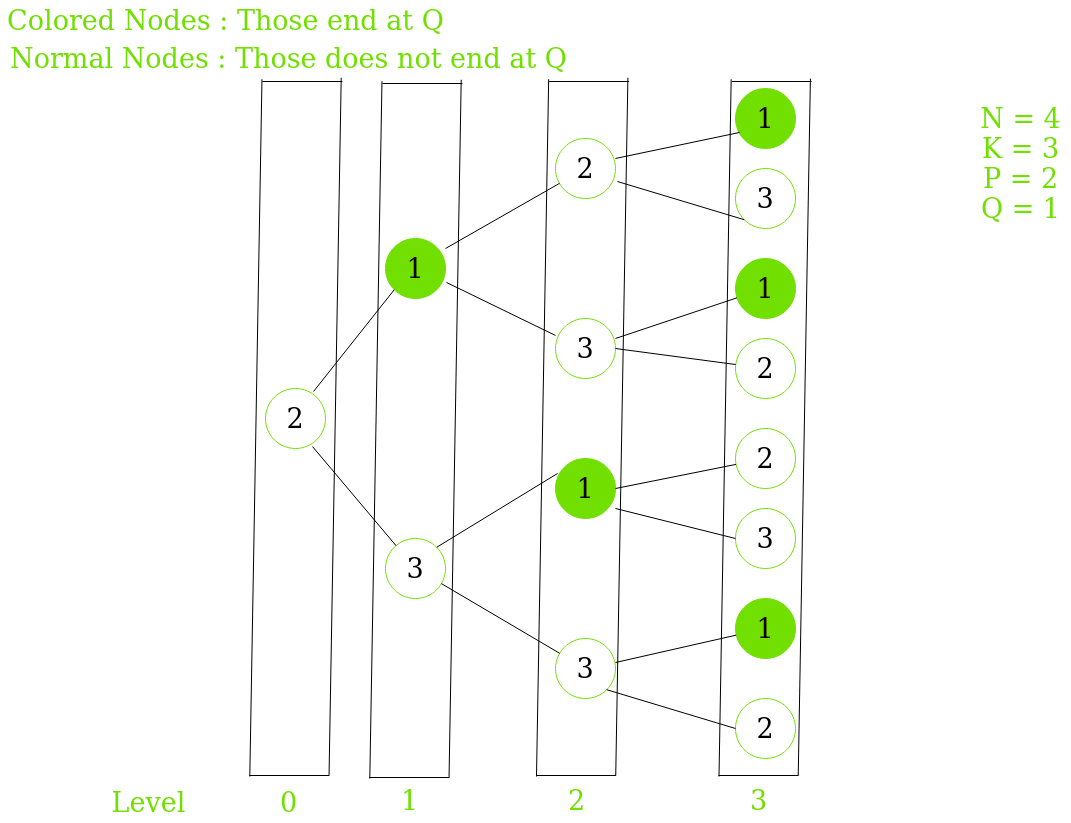# Number of ways to arrange N numbers which are in a range from 1 to K under given constraints.

Given Four integers N, K, P and Q. The task is to calculate the number of ways to arrange N numbers which are in a range from 1 to K such that the first number is P, the last number is Q and no two adjacent numbers are consecutive.

Examples:

```Input:  N = 4, K = 3, P = 2, Q = 3
Output: 3
Explanation:
For N=4, K=3, P=2, Q=3,
ways are [2, 1, 2, 3], [2, 3, 1, 3], [2, 3, 2, 3]

Input:  N = 5, K = 3, P = 2, Q = 1
Output: 5
```

## Recommended: Please try your approach on {IDE} first, before moving on to the solution.

Approach: The idea is to use Dynamic Programming to solve this problem.

• Let’s try to understand this by taking an example, N = 4, K = 3, P = 2, Q = 1.
We will observe all possible arrangements staring from P and try to find any pattern that can be useful to apply Dynamic programming.
• Below is the image showing all possible arrangements starting from P = 2.• Let A be the array that consists of the number of nodes ending at Q at a particular level
A = { 0, 1, 1, 3 }

Let B be the array that consists of the number of nodes NOT ending at Q at a particular level
B = {1, 1, 3, 5 }

• On carefull observation it may be noted that:

1. A[i] = B[i-1]
Reason :
All the favourable nodes ( ending at Q ) will only be produced by non-favourable nodes(NOT ending at Q) of the previous level.
2. B[i] = A[i-1]*(K – 1) + B[i-1]*(K – 2)
Reason :

• For A[i-1]*(K – 1), some of the non-favourable nodes are produced by favourable nodes of the previous level, multiply by (K – 1) as each favourable node will produce K-1 non-favourable nodes
• For B[i-1]*(K – 2), rest of the non-favourable nodes are produced by non-favourable nodes of the previous level, multiply by (K-2), as one produced node is favourable, so we subtract 2 from this.

 `// C++ program to calculate Number of  ` `// ways to arrange N numbers under ` `// given constraints. ` `#include ` `using` `namespace` `std; ` ` `  `class` `element { ` `public``: ` `    ``// For favourable nodes  ` `    ``// (ending at Q) ` `    ``int` `A; ` `     `  `    ``// For Non-favourable nodes ` `    ``// (NOT ending at Q) ` `    ``int` `B; ` `}; ` ` `  `// Function to print Total number ` `// of ways ` `void` `NumberOfWays(``int` `n, ``int` `k, ``int` `p,  ` `                                ``int` `q) ` `{ ` `    ``element* dp = ``new` `element[n]; ` ` `  `    ``// If the First number and the ` `    ``// last number is same. ` `    ``if` `(p == q) { ` `        ``dp.A = 1; ` `        ``dp.B = 0; ` `    ``} ` `    ``else` `    ``{ ` `        ``dp.A = 0; ` `        ``dp.B = 1; ` `    ``} ` ` `  `    ``// DP approach to find current state  ` `    ``// with the help of previous state. ` `    ``for` `(``int` `i = 1; i < n; i++) ` `    ``{ ` `        ``dp[i].A = dp[i - 1].B; ` `        ``dp[i].B = (dp[i - 1].A * (k - 1)) ` `                 ``+ (dp[i - 1].B * (k - 2)); ` `    ``} ` `     `  `    ``cout << dp[n - 1].A << endl; ` ` `  `    ``return``; ` `} ` ` `  `// Driver code ` `int` `main() ` `{ ` `    `  `   ``int` `N = 5; ` `   ``int` `K = 3; ` `   ``int` `P = 2; ` `   ``int` `Q = 1; ` `     `  `   ``// Function call ` `   ``NumberOfWays(N, K, P, Q); ` `} ` `    `

Output:

```5
```

Time Complexity: O(N).My Personal Notes arrow_drop_upCheck out this Author's contributed articles.

If you like GeeksforGeeks and would like to contribute, you can also write an article using contribute.geeksforgeeks.org or mail your article to contribute@geeksforgeeks.org. See your article appearing on the GeeksforGeeks main page and help other Geeks.

Please Improve this article if you find anything incorrect by clicking on the "Improve Article" button below.